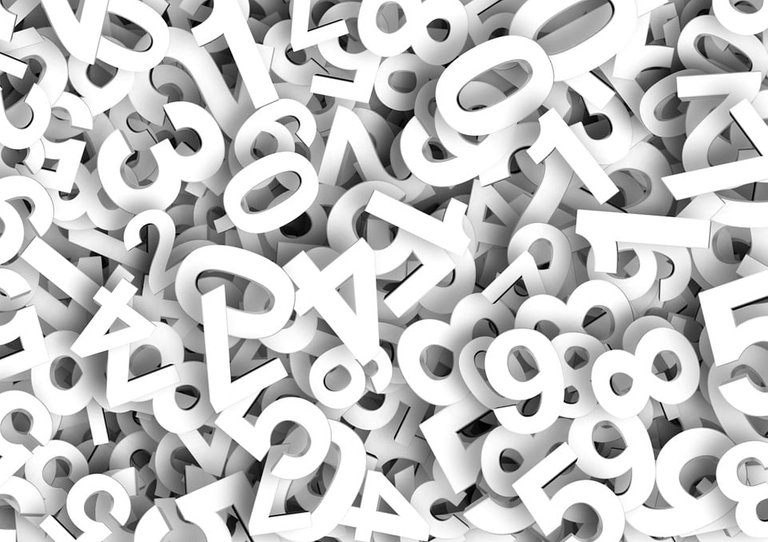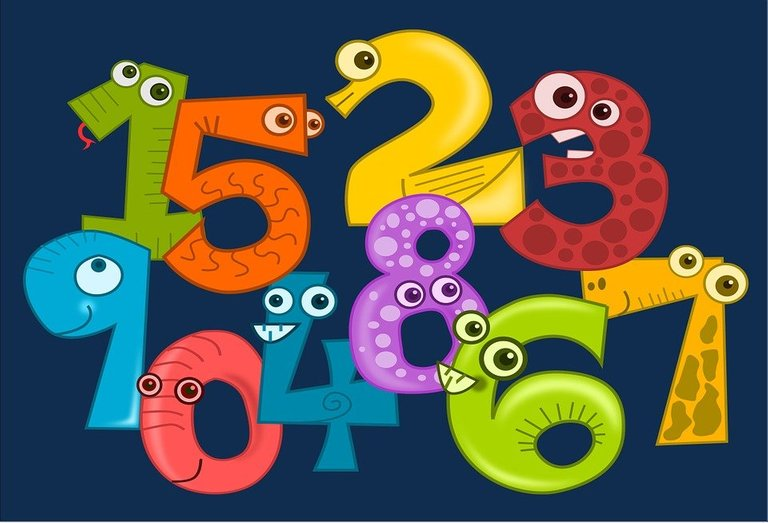Hi there. In this short math post I cover the concept of Harshad numbers. I came across this topic recently in my work. These numbers can also be called Niven numbers.Pixabay Image Source

## Sum Of Digits

From the number theory field of mathematics there are cases where you have the sum of digits. The term sum refers to the total of numbers from additions. Digits refers to a number from 0 to 9 that make up larger numbers.

For a number like 78 the digits that make up 78 are 7 and 8. The sum of the digits would be `7 + 8 = 15`.

With a larger number like 27418 the digits are 2, 7, 4, 1 and 8. The sum of the digits are `2 + 7 + 4 + 1 + 8 = 22`.Pixabay Image Source

## What Is A Harshad Number?

A Harshad number is a positive whole number (natural number) where it is divisible by the sum of its digits. That is the Harshad number can be divided by the total of its digits.

Example One

The sum of the digits in 25 is `2 + 5 = 7`. Check if 25 is divisible by 7. Twenty-five is not a part of the seven times table. It is not divisible by 7. For this question, 25 is not a Harshad number.

Example Two

Ten would be a Harshad number. The number ten has the sum of the digits as 1. Ten is divisible by one so it is a Harshad number.

You can generalize as say that powers of 10 such as 10, 100, 1000, 10000, etc. are Harshad numbers.

Example Three

Determine the sum of the digits of 777 first. This would be `7 + 7 + 7 = 21`. See if the number 777 can be divided by 21. You can use a calculator or do long division and check for no remainder by hand. Seven hundred seventy-seven can be divided by 21 as `777 ÷ 21 = 37`. The number 777 is a Harshad number.Pixabay Image Source

Posted with STEMGeeks

0
0
0.000